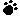Please review your test results by moving cursor to answer sheet icon.1.A)
B)
C)
D)

Your answer=, Correct answer=,

2.Which of the following statements is correct?

A) A collection of unrelated programs can constitute a portfolio.
B) The scope of a portfolio is typically smaller than that of a program.
C) A program is a group of unrelated projects.
D) A program need not consist of projects.Your answer=B, Correct answer=, Work Sheet:

3.A)
B)
C)
D)

Your answer=, Correct answer=,

4.A)
B)
C)
D)

Your answer=, Correct answer=,

5.A)
B)
C)
D)

Your answer=, Correct answer=,